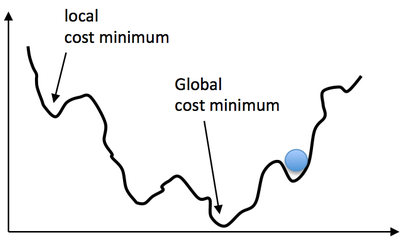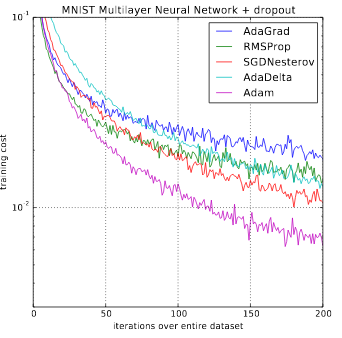This article covers a sublime explanation and a simple example of Vanilla Gradient Descent algorithm, Stochastic Gradient Descent, Momentum Optimizer, and Adam Optimizer in which RMSProp is also explained
TKTejas Khare24.00
Apr 30, 2021Optimization Methods are one of the vital aspects of Machine Learning, Deep Learning, and also just Neural Networks. For instance, a high accuracy classifier depends on the weights 'W' and bias 'b' values to obtain a minimum loss after its training. Optimization is like a driver for neural networks that enable them to learn from the data fed to the network. In simple terms, Optimization is responsible to learn the patterns from the data and predict accordingly which heavily resides on the loss factor.

Initializing the W and b parameters with random values, in the beginning, will be a good option. Actually, No, it won't be the only option and definitely not the best one. The reasons are

1. Modal Loss - It is nothing but while training, your network focuses more on some classes and performs very badly for others.
2. Even if the above option works, it will surely take a huge amount of time to complete it because modern networks contain at least a million neurons. It is not such a good idea to wait and hope that W and b will get those perfect values and we can move on.

Hence, to skip these problems, defining an optimization algorithm would be fruitful. Let us see some optimization algorithms for machine learning.

Contents of the article

2. Momentum Based
3. Nesterov Momentum
5. Comparison of the optimizers

It has two implementations

1. The standard Vanilla Implementation
2. The optimized Stochastic Gradient Descent (SGD)

### The standard Vanilla methodLet's take the above image for our reference, you can see there are multiple local minima and only one global minimum. The network needs to get to the global minima to produce the best results. To get there, we need to define a function that takes W and b to get there as it cannot just go there because it does not exist initially.

Each position on the loss line corresponds to some value of W and b and our goal is to try different values of W and b, get their loss, and take another step towards the next lower loss value. Below is an implementation for the standard Vanilla method Implementation Code

# The loop will start at x=1 for our example
i = 1
# Learning rate (Using adaptive learning rate like exponential decay for learning rate will help in complex neural networks)
rate = 0.002
#This tells us the threshold to stop the algorithm
precision = 0.000001
previous_step_size = 1
max_iters = 100000
iters = 0
# This is defined gradient function (not necessarily this is the best one)
# Using lambda in general can also speed up the process.
gd = lambda x: 2*x + 5

2. Start the loop

it_list = []
gd_list = []
while previous_step_size > precision and iters < max_iters:
prev_x = i
i = i - rate * gd(prev_x)
# Compute the difference and iterate
previous_step_size = abs(i - prev_x)
iters = iters+1
print("Iteration",iters,"\nX value is",i) #Print iterations

print("The local minimum occurs at", i)


3. Get the results

Below is an image that shows the results of the last few iterations and the final result.plt.plot(it_list, gd_list)The drawback of the 'vanilla' method is that it is very slow for bigger datasets and as I said earlier, we can't sit and hope that it would finish at some point.

The difference here is that the algorithm updates W and b on a small batch of data, ie. you can send a collection of the data into a single iteration and for the next iteration, you can send the next batch, and so on.

"Won't this predict some noisy values because it is not iterating over each data?"

Yes, it will produce noisy predictions, but the advantage here is the number of iteration can be increased that helps the network to learn efficiently.

Psuedo Code

i = 0
# Here i is just a counter which is passed to next_training_batch() function to provide batches.
while True:
batch_for_iteration = next_training_batch(data, i)
gd = batch_for_iteration.T.(batch_error)
# Here we keep updating the W for the optimal values
W += -alpha * gd
iterate i



## 2. Momentum based optimization

Gradient descent algorithm has a static or fixed-function to update the weights, which might lead to noisy updates. Earlier, I mentioned adaptive learning rates that slow down the rate of descent of the curve in the final epochs. But do not get confused with adaptive optimization. This can be beneficial for obtaining faster convergence.

It uses exponentially weighted averages of gradients from the previous iteration that smoothens the convergence, also stabilizing it.

### gd = beta * gd + (1 - beta) * l                                                     (1)

where gd is the aggregate of previous gradients

Pseudo Code

Object_function(x)
#initialize
w = 0
while True:
gd = c * gd + (1 - c) * gradient_of_object_function(x)
old_w = w
w = w - q * gd
if old_w == w:
break

## 3. Nesterov Momentum Optimizer

Nesterov Momentum Optimizer is a subset of normal momentum optimizer. The only difference is that instead of the parameter 'l', an 'l' of an intermediate position is used to compute the weighted average.where is the gradient of the loss function 'l' and learning rate 'n' and is the weights and biases (here it is l). The intermediate position in the first equation in the above image is

The Adam optimizer is like an extension of SGD, where the learning rate remains constant. In Adam, however, the learning rate is not constant and is adapted as the network converges. Something about its name, Adam is derived from Adaptive Moment Estimation.

It is highly effective with a huge amount of data and parameters as it requires a lot less memory. But first, you will need to know about another optimization method to get the gist of Adam's function.

### RMSProp

RMSProp actually improves the result of AdaGrad. In short, the AdaGrad optimization algorithm just uses variable learning rates or the learning rate slows down and is only applicable for scarce or infrequent data. Root Mean Square Propagation is an adaptive learning algorithm that uses the square of the gradient of loss. The formula is -

### gds= beta * gds + (1 - beta) * (l ** 2)                                      (2)

where GDS is the sum of squares of previous gradients

Adam is a combination of both momentum and RMSProp and gets the strengths of these two and improves the optimization. Adam is often used for large amounts of data in which classification, detection, or even generation is required. The formula we get after combining equations 1) and 2) is Adam's equation -

### gds= beta2 * gds + (1 - beta2) * (l ** 2)

where beta1 and beta2 are decay rates for respective average gradients.

## 5. Comparison of optimizer algorithmsThe above comparison graph experiments on the MNIST dataset and as you can see, Adam overpowers the other optimizers by converging the network faster and efficiently. There is clearly a significant difference between Nesterov and Adam, given Nesterov is also a highly effective algorithm.

I hope this article gave you a good insight into the optimization methods in machine learning. Keep on (machine) learning. Cheers.